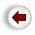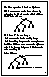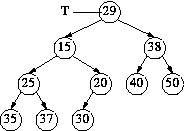### Sift DownThe DELETE operation is based on `SIFT DOWN', which, as the name suggests, is the exact opposite of SIFT UP.

SIFT DOWN starts with a value in any node. It moves the value down the tree by successively exchanging the value with the smaller of its two children. The operation continues until the value reaches a position where it is less than both its children, or, failing that, until it reaches a leaf.

Example: sift down 29 in this tree:

``

Steps:

• 29 is compared with 15 and 38. Exchange 29 and 15 and repeat.
• 29 is compared with 25 and 20. Exchange 29 and 20 and repeat.
• 29 is compared with 30. 29 is smaller, so stop.
Note: The tree's shape does not change. if it was a complete tree to start with, it will still be complete when the operation is done.

Complexity = O(height) = O(logN)